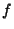## Isometry

A Bijective Map between two Metric Spaces that preserves distances, i.e.,whereis the Map andis the Distance function.

An isometry of the Plane is a linear transformation which preserves length. Isometries include Rotation, Translation, Reflection, Glides, and the Identity Map. If an isometry has more than one Fixed Point, it must be either the identity transformation or a reflection. Every isometry of period two (two applications of the transformation preserving lengths in the original configuration) is either a reflection or a half turn rotation. Every isometry in the plane is the product of at most three reflections (at most two if there is a Fixed Point). Every finite group of isometries has at least one Fixed Point.

See also Distance, Euclidean Motion, Hjelmslev's Theorem, Length (Curve), Reflection, Rotation, Translation

References

Gray, A. Isometries of Surfaces.'' §13.2 in Modern Differential Geometry of Curves and Surfaces. Boca Raton, FL: CRC Press, pp. 255-258, 1993.